# Sliding-cum-rotational motion along an inclined plane

Jump to: navigation, search
This article discusses a scenario/arrangement whose statics/dynamics/kinematics can be understood using the ideas of classical mechanics.
View other mechanics scenarios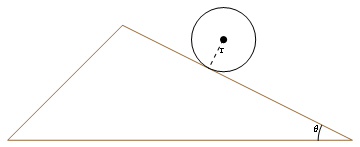A rolling-capable object on an incline, cross sectional view. The object may be a cylinder or sphere, among other possibilities.

The scenario here is a dry body placed on a fixed dry inclined plane with an angle of inclination$\theta$ with the horizontal, where the body is capable of rolling along the flat incline. This includes the following possibilities for the body: solid cylinder, hollow cylinder, cylindrical annulus, solid sphere, hollow sphere. We assume that the center of rotation is also the center of the object (this happens in all the above specific examples assuming uniform density).

The extremes are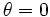$\theta = 0$ (whence, the plane is horizontal) and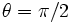$\theta = \pi/2$ (whence, the plane is vertical).

## Basic components of force and torque diagrams

This part of the analysis is the same as for sliding motion along an inclined plane

A good way of understanding the force diagram is using the coordinate axes as the axis along the inclined plane and normal to the inclined plane. For simplicity, we will assume a two-dimensional situation, with no forces acting along the horizontal axis that is part of the inclined plane (in our pictorial representation, this no action axis is the axis perpendicular to the plane).

### Taking components of the gravitational force

KEY FORCE CONCEPT (GRAVITY): For an object of mass$m$ close to the surface of the earth, gravity acts in a downward direction (toward the center of the earth) and has magnitude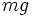$mg$, regardless of the presence or absence of other forces and regardless of the object's motion, velocity, or acceleration. Thus, the component-taking of the gravitational force for the mass$m$ does not depend on whether the mass slides, rolls, or rotates, or the nature of other forces being exerted on this mass.

The most important thing for the force diagram is understanding how the gravitational force, which acts vertically downward on the block, splits into components along and perpendicular to the incline. The component along the incline is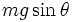$mg\sin \theta$ and the component perpendicular to the incline is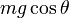$mg\cos \theta$. The process of taking components is illustrated in the adjacent figure.

### Component perpendicular to the inclined plane

KEY FORCE CONCEPT (ACTION-REACTION): Just because two forces are equal in magnitude and opposite in direction does not imply that they form an action-reaction pair in the sense of Newton's third law of motion. An action-reaction pair is a pair occurs between two bodies that exert forces on each other, not for a pair of forces both acting on the same body.$N$ and$mg\cos \theta$, though equal and opposite, do not form an action-reaction pair in the sense of Newton's third law. Rather, they are equal and opposite because there is no net acceleration along the normal direction.

In this case, assuming a stable surface of contact, and that the inclined plane does not break under the weight of the block, and no other external forces, we get the following equation from Newton's first law of motion applied to the axis perpendicular to the inclined plane: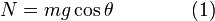$\! N = mg \cos \theta\qquad\qquad(1)$

where$N$ is the normal force between the block and the inclined plane,$m$ is the mass of the block, and$g$ is the acceleration due to gravity.$N$ acts inward on the inclined plane and outward on the block. Some observations:

Value/change in value of$\theta$ Value of$N$ Comments$\theta = 0$ (horizontal plane)$N = mg$ The normal force exerted on a horizontal surface equals the mass times the acceleration due to gravity, which we customarily call the weight.$\theta = \pi/2$ (vertical plane)$N = 0$ The block and the inclined plane are barely in contact and hardly pressed together.$\theta$ increases from$0$ to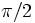$\pi/2$$N$ reduces from$mg$ to$0$. The derivative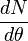$\frac{dN}{d\theta}$ is$-mg\sin \theta$ The force pressing the block and the inclined plane reduces as the slope of the incline increases.

For simplicity, we ignore the cases$\theta = 0$ and$\theta = \pi/2$ unless specifically dealing with them.

### The rest of the analysis

This part of the analysis differs from that for sliding motion along an inclined plane because, for the purposes of determining slippage, we care, not about the overall acceleration and velocity of the body, but about the acceleration and velocity at the area of contact.

Suppose$a$ is the acceleration of the object measured positive down the inclined plane, and$\alpha$ is the angle of rotation, measured positive in the direction that it would be if the object were rolling down. Since there are multiple cases, and the cases are qualitatively different, we consider the cases separately.

## The rolling down case

If the object is rolling down, we have the relation:$\! a = r\alpha\qquad\qquad (2)$

This arises as follows: the relative velocity at the region of contact is zero, hence, differentiating, the relative acceleration is zero. But the relative acceleration at the region of contact is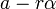$a - r\alpha$. Thus, we get$a = r\alpha$.

Further, we have the following equation for the component of force diagram down the inclined plane:$\! mg\sin \theta - f_s = ma\qquad \qquad (3)$

Finally, we have the following torque equation about the center of the object:$\! f_sr = I\alpha \qquad\qquad(4)$

This torque equation arises by the following reasoning: of the three forces$mg$,$N$, and$f_s$, the first two act through the center of the object, hence$f_s$ is the only contributor to a torque, and it acts at a distance$r$ from the center.

Finally, we have the constraint:$\! f_s \le \mu_sN \qquad\qquad(5)$

Substituting (2) in (4) gives: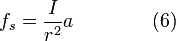$\! f_s = \frac{I}{r^2}a \qquad\qquad(6)$

Adding (3) and (6), we get: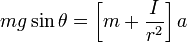$\! mg\sin \theta = \left[m + \frac{I}{r^2}\right]a$

Dividing both sides by$m$, we obtain:$\! g\sin \theta = \left[1 + \frac{I}{mr^2}\right]a$

Simplifying, we get:$\! a = \frac{g \sin \theta}{1 + \frac{I}{mr^2}}\qquad\qquad(7)$

Using (2), we get:$\! \alpha = \frac{g \sin \theta}{r + \frac{I}{mr}}\qquad\qquad(8)$

Note that we have still not dealt with the constraint arising from (5). We do so now. Combining (5) with (1) (the component of the force diagram perpendicular to the inclined plane) we get: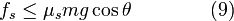$\! f_s \le \mu_smg\cos \theta\qquad\qquad(9)$

Also, combining (3) and (7), we get:$\! f_s = \frac{mg\sin \theta(I/mr^2)}{1 + (I/mr^2)}\qquad\qquad(10)$

Combining (9) and (10), we obtain that:$\! mg\sin\theta(I/mr^2) \le \mu_smg\cos \theta[1 + (I/mr^2)]$

The$m$ and$g$ cancel out, and we get: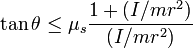$\! \tan \theta \le \mu_s\frac{1 + (I/mr^2)}{(I/mr^2)}$

Or equivalently:$\! \theta \le \tan^{-1}[\mu_s\frac{1 + (I/mr^2)}{(I/mr^2)}]$

The upshot is that:

• If$\theta \le \tan^{-1}[\mu_s\frac{1 + (I/mr^2)}{(I/mr^2)}]$ then rolling motion is possible, with$\! a = \frac{g \sin \theta}{1 + \frac{I}{mr^2}}$,$\alpha = a/r$, and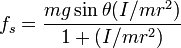$f_s = \frac{mg\sin \theta(I/mr^2)}{1 + (I/mr^2)}$.
• If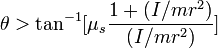$\theta > \tan^{-1}[\mu_s\frac{1 + (I/mr^2)}{(I/mr^2)}]$, then rolling motion is not possible.

We now consider special cases of various kinds of objects, and for each, we determine the maximum value$\theta$ above which rolling is not possible, as well as the value of$a$ in the general and limiting case. Note that$\alpha = a/r$ in each case. Also note the contrast with sliding: in the case of sliding, the maximum angle for which there is no slippage is the angle of friction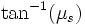$\tan^{-1}(\mu_s)$, whereas here the maximum angle for rolling (which means no slippage) is always bigger than that.

Object$I/mr^2$$(\tan \theta)/\mu_s$ for largest$\theta$ allowing rolling$a$ assuming rolling$a$ for largest$\theta$ allowing rolling
General$q$ (say)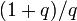$(1 + q)/q$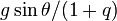$g\sin\theta/(1 + q)$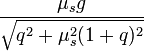$\frac{\mu_sg}{\sqrt{q^2 + \mu_s^2(1 + q)^2}}$
Hollow cylinder 1 2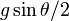$g\sin \theta/2$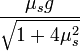$\frac{\mu_sg}{\sqrt{1 + 4\mu_s^2}}$
Hollow sphere 2/3 5/2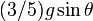$(3/5)g\sin \theta$$\frac{3\mu_sg}{\sqrt{4 + 25\mu_s^2}}$
Solid cylinder 1/2 3$(2/3)g\sin \theta$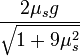$\frac{2\mu_sg}{\sqrt{1 + 9\mu_s^2}}$
Solid sphere 2/5 7/2$(5/7)g\sin \theta$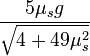$\frac{5\mu_sg}{\sqrt{4 + 49\mu_s^2}}$

Here are the graphs (in radians and degrees) plotting the maximum angles$\theta$ as a function of$\mu_s$. The lowest line is$\tan^{-1}(\mu_s)$, representing the angle of friction, and all the other lines represent the various rolling possibilities, from low to high: hollow cylinder, hollow sphere, solid cylinder, solid sphere.